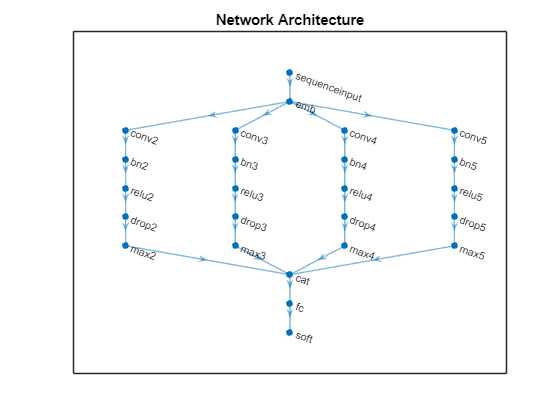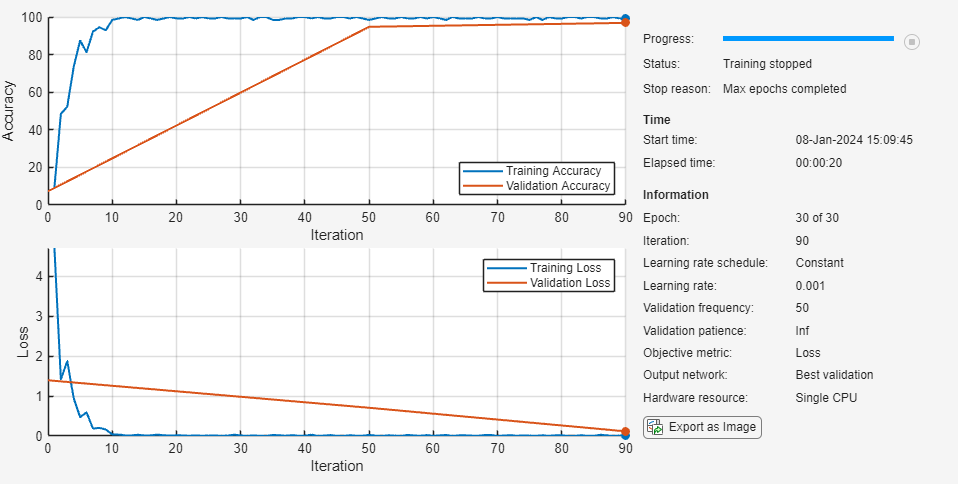## Classify Text Data Using Convolutional Neural Network

This example shows how to classify text data using a convolutional neural network.

To classify text data using convolutions, you must convert the text data into images. To do this, pad or truncate the observations to have constant length S and convert the documents into sequences of word vectors of length C using a word embedding. You can then represent a document as a 1-by-S-by-C image (an image with height 1, width S, and C channels).

To convert text data from a CSV file to images, create a `tabularTextDatastore` object. The convert the data read from the `tabularTextDatastore` object to images for deep learning by calling `transform` with a custom transformation function. The `transformTextData` function, listed at the end of the example, takes data read from the datastore and a pretrained word embedding, and converts each observation to an array of word vectors.

This example trains a network with 1-D convolutional filters of varying widths. The width of each filter corresponds the number of words the filter can see (the n-gram length). The network has multiple branches of convolutional layers, so it can use different n-gram lengths.

Load the pretrained fastText word embedding. This function requires the Text Analytics Toolbox™ Model for fastText English 16 Billion Token Word Embedding support package. If this support package is not installed, then the function provides a download link.

`emb = fastTextWordEmbedding;`

Create a tabular text datastore from the data in `weatherReportsTrain.csv`. Read the data from the `"event_narrative"` and `"event_type"` columns only.

```filenameTrain = "weatherReportsTrain.csv"; textName = "event_narrative"; labelName = "event_type"; ttdsTrain = tabularTextDatastore(filenameTrain,'SelectedVariableNames',[textName labelName]);```

Preview the datastore.

```ttdsTrain.ReadSize = 8; preview(ttdsTrain)```
```ans=8×2 table event_narrative event_type ___________________________________________________________________________________________________________________________________________________________________________________________________ _____________________ {'Large tree down between Plantersville and Nettleton.' } {'Thunderstorm Wind'} {'One to two feet of deep standing water developed on a street on the Winthrop University campus after more than an inch of rain fell in less than an hour. One vehicle was stalled in the water.'} {'Heavy Rain' } {'NWS Columbia relayed a report of trees blown down along Tom Hall St.' } {'Thunderstorm Wind'} {'Media reported two trees blown down along I-40 in the Old Fort area.' } {'Thunderstorm Wind'} {'A few tree limbs greater than 6 inches down on HWY 18 in Roseland.' } {'Thunderstorm Wind'} {'Awning blown off a building on Lamar Avenue. Multiple trees down near the intersection of Winchester and Perkins.' } {'Thunderstorm Wind'} {'Tin roof ripped off house on Old Memphis Road near Billings Drive. Several large trees down in the area.' } {'Thunderstorm Wind'} {'Powerlines down at Walnut Grove and Cherry Lane roads.' } {'Thunderstorm Wind'} ```

Create a custom transform function that converts data read from the datastore to a table containing the predictors and the responses. The `transformTextData` function, listed at the end of the example, takes the data read from a `tabularTextDatastore` object and returns a table of predictors and responses. The predictors are 1-by-`sequenceLength`-by-C arrays of word vectors given by the word embedding `emb`, where C is the embedding dimension. The responses are categorical labels over the classes in `classNames`.

Read the labels from the training data using the `readLabels` function, listed at the end of the example, and find the unique class names.

```labels = readLabels(ttdsTrain,labelName); classNames = unique(labels); numObservations = numel(labels);```

Transform the datastore using `transformTextData` function and specify a sequence length of 100.

```sequenceLength = 100; tdsTrain = transform(ttdsTrain, @(data) transformTextData(data,sequenceLength,emb,classNames))```
```tdsTrain = TransformedDatastore with properties: UnderlyingDatastore: [1×1 matlab.io.datastore.TabularTextDatastore] Transforms: {@(data)transformTextData(data,sequenceLength,emb,classNames)} IncludeInfo: 0 ```

Preview the transformed datastore. The predictors are 1-by-S-by-C arrays, where S is the sequence length and C is the number of features (the embedding dimension). The responses are the categorical labels.

`preview(tdsTrain)`
```ans=8×2 table predictors responses __________________ _________________ {1×100×300 single} Thunderstorm Wind {1×100×300 single} Heavy Rain {1×100×300 single} Thunderstorm Wind {1×100×300 single} Thunderstorm Wind {1×100×300 single} Thunderstorm Wind {1×100×300 single} Thunderstorm Wind {1×100×300 single} Thunderstorm Wind {1×100×300 single} Thunderstorm Wind ```

Create a transformed datastore containing the validation data in `weatherReportsValidation.csv` using the same steps.

```filenameValidation = "weatherReportsValidation.csv"; ttdsValidation = tabularTextDatastore(filenameValidation,'SelectedVariableNames',[textName labelName]); tdsValidation = transform(ttdsValidation, @(data) transformTextData(data,sequenceLength,emb,classNames))```
```tdsValidation = TransformedDatastore with properties: UnderlyingDatastore: [1×1 matlab.io.datastore.TabularTextDatastore] Transforms: {@(data)transformTextData(data,sequenceLength,emb,classNames)} IncludeInfo: 0 ```

### Define Network Architecture

Define the network architecture for the classification task.

The following steps describe the network architecture.

• Specify an input size of 1-by-S-by-C, where S is the sequence length and C is the number of features (the embedding dimension).

• For the n-gram lengths 2, 3, 4, and 5, create blocks of layers containing a convolutional layer, a batch normalization layer, a ReLU layer, a dropout layer, and a max pooling layer.

• For each block, specify 200 convolutional filters of size 1-by-N and pooling regions of size 1-by-S, where N is the n-gram length.

• Connect the input layer to each block and concatenate the outputs of the blocks using a depth concatenation layer.

• To classify the outputs, include a fully connected layer with output size K, a softmax layer, and a classification layer, where K is the number of classes.

First, in a layer array, specify the input layer, the first block for unigrams, the depth concatenation layer, the fully connected layer, the softmax layer, and the classification layer.

```numFeatures = emb.Dimension; inputSize = [1 sequenceLength numFeatures]; numFilters = 200; ngramLengths = [2 3 4 5]; numBlocks = numel(ngramLengths); numClasses = numel(classNames);```

Create a layer graph containing the input layer. Set the normalization option to `'none'` and the layer name to `'input'`.

```layer = imageInputLayer(inputSize,'Normalization','none','Name','input'); lgraph = layerGraph(layer);```

For each of the n-gram lengths, create a block of convolution, batch normalization, ReLU, dropout, and max pooling layers. Connect each block to the input layer.

```for j = 1:numBlocks N = ngramLengths(j); block = [ convolution2dLayer([1 N],numFilters,'Name',"conv"+N,'Padding','same') batchNormalizationLayer('Name',"bn"+N) reluLayer('Name',"relu"+N) dropoutLayer(0.2,'Name',"drop"+N) maxPooling2dLayer([1 sequenceLength],'Name',"max"+N)]; lgraph = addLayers(lgraph,block); lgraph = connectLayers(lgraph,'input',"conv"+N); end```

View the network architecture in a plot.

```figure plot(lgraph) title("Network Architecture")```Add the depth concatenation layer, the fully connected layer, the softmax layer, and the classification layer.

```layers = [ depthConcatenationLayer(numBlocks,'Name','depth') fullyConnectedLayer(numClasses,'Name','fc') softmaxLayer('Name','soft') classificationLayer('Name','classification')]; lgraph = addLayers(lgraph,layers); figure plot(lgraph) title("Network Architecture")```Connect the max pooling layers to the depth concatenation layer and view the final network architecture in a plot.

```for j = 1:numBlocks N = ngramLengths(j); lgraph = connectLayers(lgraph,"max"+N,"depth/in"+j); end figure plot(lgraph) title("Network Architecture")```### Train Network

Specify the training options:

• Train for 10 epochs with a mini-batch size of 128.

• Do not shuffle the data because the datastore is not shuffleable.

• Validate the network at each epoch by setting the validation frequency to the number of iterations per epoch.

• Display the training progress plot and suppress the verbose output.

```miniBatchSize = 128; numIterationsPerEpoch = floor(numObservations/miniBatchSize); options = trainingOptions('adam', ... 'MaxEpochs',10, ... 'MiniBatchSize',miniBatchSize, ... 'Shuffle','never', ... 'ValidationData',tdsValidation, ... 'ValidationFrequency',numIterationsPerEpoch, ... 'Plots','training-progress', ... 'Verbose',false);```

Train the network using the `trainNetwork` function.

`net = trainNetwork(tdsTrain,lgraph,options);`### Test Network

Create a transformed datastore containing the held-out test data in `weatherReportsTest.csv`.

```filenameTest = "weatherReportsTest.csv"; ttdsTest = tabularTextDatastore(filenameTest,'SelectedVariableNames',[textName labelName]); tdsTest = transform(ttdsTest, @(data) transformTextData(data,sequenceLength,emb,classNames))```
```tdsTest = TransformedDatastore with properties: UnderlyingDatastore: [1×1 matlab.io.datastore.TabularTextDatastore] Transforms: {@(data)transformTextData(data,sequenceLength,emb,classNames)} IncludeInfo: 0 ```

Read the labels from the `tabularTextDatastore`.

```labelsTest = readLabels(ttdsTest,labelName); YTest = categorical(labelsTest,classNames);```

Make predictions on the test data using the trained network.

`YPred = classify(net,tdsTest);`

Calculate the classification accuracy on the test data.

`accuracy = mean(YPred == YTest)`
```accuracy = 0.8795 ```

### Functions

The `readLabels` function creates a copy of the `tabularTextDatastore` object `ttds` and reads the labels from the `labelName` column.

```function labels = readLabels(ttds,labelName) ttdsNew = copy(ttds); ttdsNew.SelectedVariableNames = labelName; tbl = readall(ttdsNew); labels = tbl.(labelName); end```

The `transformTextData` function takes the data read from a `tabularTextDatastore` object and returns a table of predictors and responses. The predictors are 1-by-`sequenceLength`-by-C arrays of word vectors given by the word embedding `emb`, where C is the embedding dimension. The responses are categorical labels over the classes in `classNames`.

```function dataTransformed = transformTextData(data,sequenceLength,emb,classNames) % Preprocess documents. textData = data{:,1}; textData = lower(textData); documents = tokenizedDocument(textData); % Convert documents to embeddingDimension-by-sequenceLength-by-1 images. predictors = doc2sequence(emb,documents,'Length',sequenceLength); % Reshape images to be of size 1-by-sequenceLength-embeddingDimension. predictors = cellfun(@(X) permute(X,[3 2 1]),predictors,'UniformOutput',false); % Read labels. labels = data{:,2}; responses = categorical(labels,classNames); % Convert data to table. dataTransformed = table(predictors,responses); end```